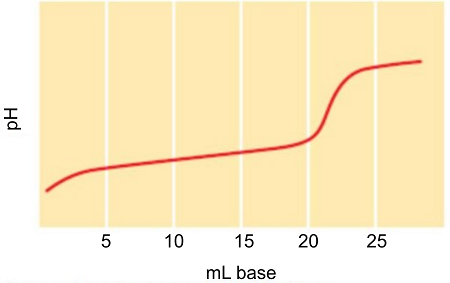# Consider the titration of a generic weak acid HA with a strong base that gives the following...

## Question:

Consider the titration of a generic weak acid HA with a strong base that gives the following titration curve:On the curve indicate the points that correspond to the following.

a. the equivalence point

b. the maximum buffering region

c. {eq}\text{pH}=\text{p}{{K}_{\text{a}}} {/eq}

d. pH depends only on {eq}\left[ \text{HA} \right] {/eq}

e. pH depends only on {eq}\left[ {{\text{A}}^{-}} \right] {/eq}

f. pH depends only on the amount of excess strong base added

## Weak acid

Acids that are only partially ionized when dissolved in water are considered weak acids. Examples of weak acids are acetic acid, {eq}CH_3COOH {/eq} and nitrous acid, {eq}HNO_2 {/eq}. Weak acids produce strong conjugate bases. This is the reason why in the titration of a weak acid with a strong base, the pH of the solution at the equivalence point is above 7.00.